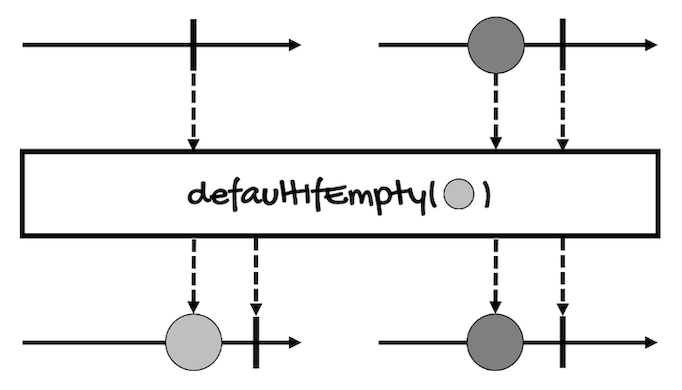# Branching on Empty Sequences

In previous modules and lessons, I mentioned that in some cases, an `if` statement inside a `map` or `flatMap` operator can be replaced by another operator.

Well, in addition to filter and other operators that can include or exclude elements based on condition (`Predicate`), another popular conditional operation is to return something else when a sequence/stream/collection is empty.

For this, there are two operators that can help us:

## defaultIfEmpty

This operator returns the provided single value if the sequence is empty or completes without values:

``````// For Mono
Mono<T> defaultIfEmpty(T defaultV)

// For Flux
Flux<T> defaultIfEmpty(T defaultV)
``````

Notice this operator returns the value wrapped in either a `Mono` or a `Flux` (depending on the `Publisher` we’re using).

This is the marble diagram of this operator (it’s the same for `Mono` and `Flux`):Here’s an example:

``````Flux<Integer> integerFlux =
Flux.just(1, 2, 3, 4, 5);

integerFlux
.filter(i -> i > 10)
.defaultIfEmpty(-1)
.subscribe(System.out::println);
``````

This is the result:

``````-1
``````

The predicate of the `filter` operator will evaluate to `false` for all the elements, so it will return an empty `Flux`. This way, the `defaultIfEmpty` operator will provide an alternative value wrapped in a `Flux` (`-1`), which will be published at the end.

If we change the predicate so at least one element evaluates to `true`:

``````Flux<Integer> integerFlux =
Flux.just(1, 2, 3, 4, 5);

integerFlux
.filter(i -> i > 4)
.defaultIfEmpty(-1)
.subscribe(System.out::println);
``````

`defaultIfEmpty` won’t change the `Flux` that `filter` returns.

Here’s the result of the above example:

``````5
``````

## switchIfEmpty

This operator switches to the given `Publisher` if the sequence is empty or completes without values:

``````// For Mono
Mono<T> switchIfEmpty(
Mono<? extends T> alternate
)

// For Flux
Flux<T> switchIfEmpty(
Publisher<? extends T> alternate
)
``````

`switchIfEmpty` is to `defaultIfEmpty` just as `flatMap` is to `map` because it takes a `Publisher` as argument instead of a simple value.

For instance, this is the marble diagram of this operator for `Mono`:Here’s an example:

``````Flux<Integer> integerFlux =
Flux.just(1, 2, 3, 4, 5);

integerFlux
.filter(i -> i > 10)
.switchIfEmpty(Flux.just(-1, 0, 99))
.subscribe(System.out::println);
``````

This is the result:

``````-1
0
99
``````

If we change the predicate so at least one element evaluates to `true`:

``````Flux<Integer> integerFlux =
Flux.just(1, 2, 3, 4, 5);

integerFlux
.filter(i -> i > 4)
.switchIfEmpty(Flux.just(-1, 0, 99))
.subscribe(System.out::println);
``````

`switchIfEmpty` won’t switch to the alternative `Flux` it provides.

Here’s the result of the above example:

``````5
``````